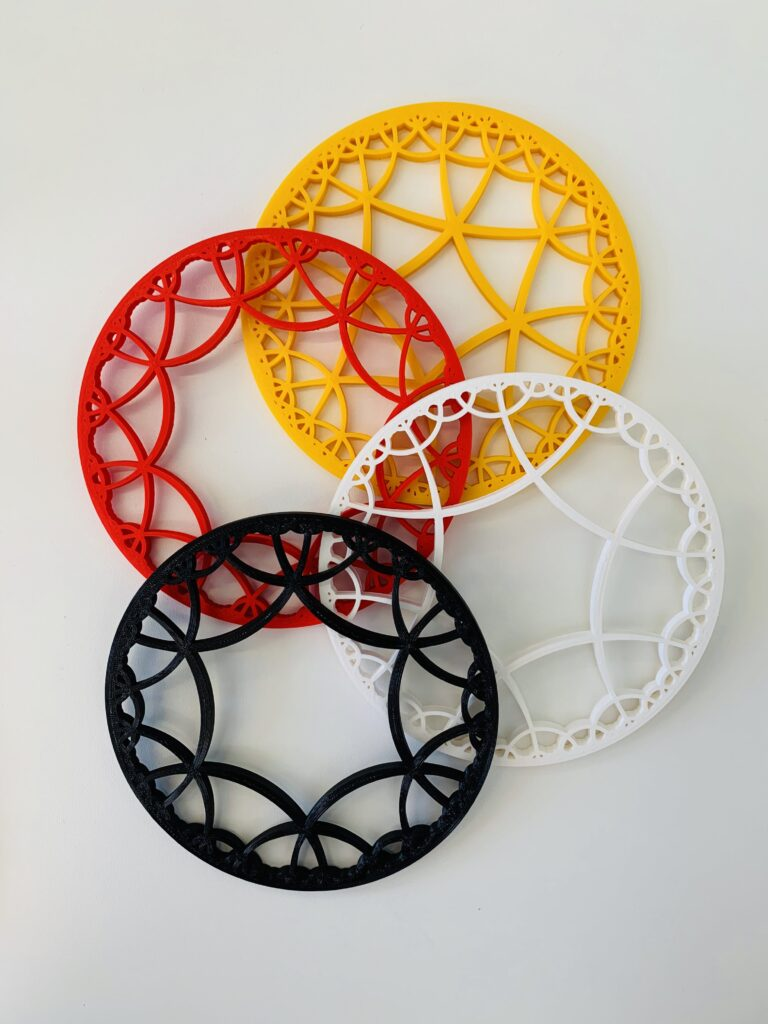## Visualizing Topology and Geometry with a Physics ConnectionWritten by Ricardo Waibel in collaboration with Donald Youmans Topology and geometry are important concepts in mathematics and physics. Especially in theories like general relativity they have a direct impact, as the spacetime description of a system is already quite…

## Indra’s PearlsWritten by Alassane Diagne and Ayşegül Peközsoy Möbius transformations are functions of the form $$T\colon \mathbb{C}\rightarrow\mathbb{C},\ T(z) = \frac{az+b}{cz+d}$$ with $$a$$, $$b$$, $$c$$, $$d$$ complex numbers. We often associate the mapping $$T$$ with the matrix $$T=\begin{pmatrix}a&b\\ c&d\end{pmatrix}$$. If you look…

## Escher in 3DWritten by Helen Freytag and Ronya Ramrath. Inspired by Escher’s experimentation with hyperbolic art (and driven by the tempting availability of 3D printers in the clearly very well-funded experimental geometry lab), this project was concerned with extrapolating and printing the…

## Delta-Slim TrianglesWritten by Deniz Aydın and Simon Heidrich. It is easy to imagine how spherical triangles are “fatter” than Euclidean ones. Just like this, hyperbolic triangles look slimmer in comparison. Gromov used this property to generalize hyperbolic spaces. Imagine δ-neighborhoods of…